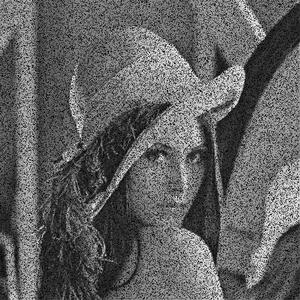Related Articles

# Add a “salt and pepper” noise to an image with Python

• Last Updated : 17 Dec, 2020

In this article, we are going to see how to add a “salt and pepper” noise to an image with Python.

Noise: Noise means random disturbance in a signal in a computer version. In our case, the signal is an image. Random disturbance in the brightness and color of an image is called Image noise.

Salt-and-pepper: It is found only in greyscale images (black and white image). As the name suggests salt (white) in pepper (black)–white spots in the dark regions or pepper (black) in salt (white)–black spots in the white regions. In other words, an image having salt-and-pepper noise will have a few dark pixels in bright regions and a few bright pixels in dark regions. Salt-and-pepper noise is also called impulse noise. It can be caused by several reasons like dead pixels, analog-to-digital conversion error, bit transmission error, etc.

Let’s see how to add salt-and-pepper noise in an image –

• Salt-and-pepper noise can only be added in a greyscale image. So, convert an image to greyscale after reading it
• Randomly pick the number of pixels to which noise is added (number_of_pixels)
• Randomly pick some pixels in the image to which noise will be added. It can be done by randomly picking x and y coordinate
• Note the random values generated must be within the range of the image dimensions. The x and y coordinates must be within the range of the image size
• Random numbers can be generated using random number generator functions like random.randint used in the code
• Color some randomly picked pixels as black setting their value to 0
• Color some randomly picked pixels as white setting their value to 255
• Save the value of the image

Below is the implementation:

## Python

 `import` `random``import` `cv2`` ` `def` `add_noise(img):`` ` `    ``# Getting the dimensions of the image``    ``row , col ``=` `img.shape``     ` `    ``# Randomly pick some pixels in the``    ``# image for coloring them white``    ``# Pick a random number between 300 and 10000``    ``number_of_pixels ``=` `random.randint(``300``, ``10000``)``    ``for` `i ``in` `range``(number_of_pixels):``       ` `        ``# Pick a random y coordinate``        ``y_coord``=``random.randint(``0``, row ``-` `1``)``         ` `        ``# Pick a random x coordinate``        ``x_coord``=``random.randint(``0``, col ``-` `1``)``         ` `        ``# Color that pixel to white``        ``img[y_coord][x_coord] ``=` `255``         ` `    ``# Randomly pick some pixels in``    ``# the image for coloring them black``    ``# Pick a random number between 300 and 10000``    ``number_of_pixels ``=` `random.randint(``300` `, ``10000``)``    ``for` `i ``in` `range``(number_of_pixels):``       ` `        ``# Pick a random y coordinate``        ``y_coord``=``random.randint(``0``, row ``-` `1``)``         ` `        ``# Pick a random x coordinate``        ``x_coord``=``random.randint(``0``, col ``-` `1``)``         ` `        ``# Color that pixel to black``        ``img[y_coord][x_coord] ``=` `0``         ` `    ``return` `img`` ` `# salt-and-pepper noise can``# be applied only to greyscale images``# Reading the color image in greyscale image``img ``=` `cv2.imread(``'lena.jpg'``,``                 ``cv2.IMREAD_GRAYSCALE)`` ` `#Storing the image``cv2.imwrite(``'salt-and-pepper-lena.jpg'``,``            ``add_noise(img))`

Output:Input image: “lena.jpg”Output image: “Salt-and-pepper-lena.jpg”

Attention geek! Strengthen your foundations with the Python Programming Foundation Course and learn the basics.

To begin with, your interview preparations Enhance your Data Structures concepts with the Python DS Course. And to begin with your Machine Learning Journey, join the Machine Learning – Basic Level Course

My Personal Notes arrow_drop_up